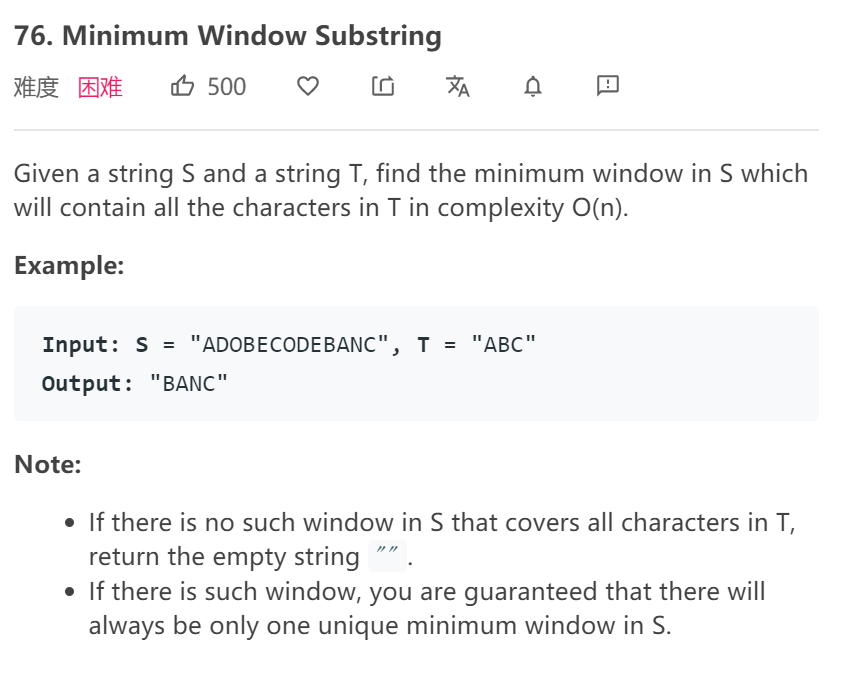# LeetCode 76/滑动窗口/双指针1.何时移动右指针（扩大窗口）？

2.何时移动左指针（缩小窗口）？

• 设置两个字典（哈希表实现），因为这里相同字符可能出现多次，而s的子串必须出现同样多次的t中出现过的字符。
• 总体思路：
1.先不断移动右指针，当window里出现了全部need里的字符的时候（覆盖了t时）。
2.这个时候开始不断移动左指针，同时更新最短窗口的起点和长度。一旦window里某个字符比need要少了，停止移动左指针，改为移动右指针。（因为答案可能是交叠出现的）
class Solution {
public:
string minWindow(string s, string t) {
unordered_map<char, int> need, window;
for (char c : t) need[c]++; //一个字符还可能出现多次？？？

int left = 0, right = 0;
int valid = 0; //为什么要设置valid? 因为字符可能重复，所以每个字符出现次数相同的时候，才能算全覆盖
// 记录最小覆盖子串的起始索引及长度
int start = 0, len = INT_MAX;
while (right < s.size()) {
// c 是将移入窗口的字符
char c = s[right]; right++;

// 进行窗口内数据的一系列更新
if (need.count(c)) { //如果是t中的字符
window[c]++;
if (window[c] == need[c]) //元素个数一样的话
valid++;
}

// 判断左侧窗口是否要收缩
while (valid == need.size()) { //当窗口内减少了一个t中的字符，我们就应该开始移动右指针了，停止移动左指针
// 在这里更新最小覆盖子串
if (right - left < len) { //区间为[left,right) 所以这里不用+1
start = left;
len = right - left;
}
// d 是将移出窗口的字符
char d = s[left++];
// 左移窗口

// 如果t内有d
if (need.count(d)) {
//有可能窗口内的这个字符比need多，所以要等到相等再减
if (window[d] == need[d]) valid--;
window[d]--;
}
}
}
// 返回最小覆盖子串
return len == INT_MAX ? "" : s.substr(start, len);
}

};©️2019 CSDN 皮肤主题: 数字20 设计师: CSDN官方博客点击重新获取扫码支付